# atomic_nucleus.ppt

24. Jul 2022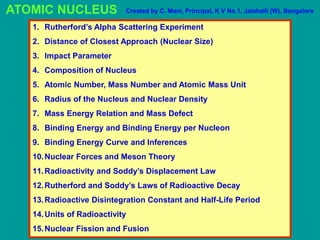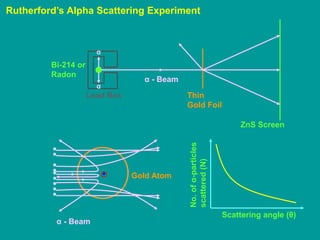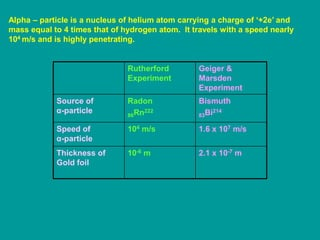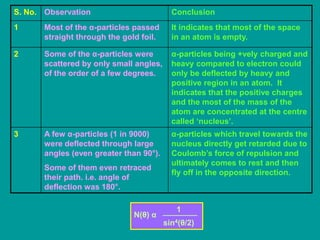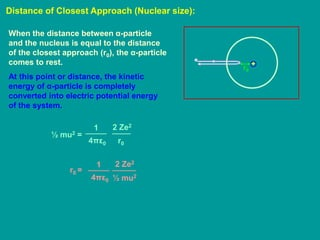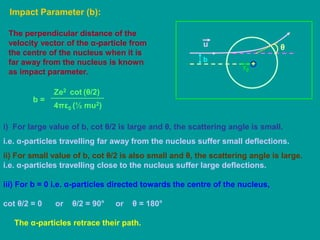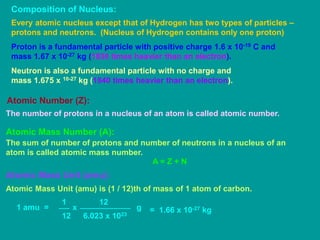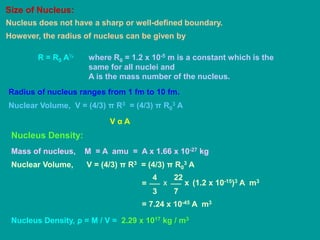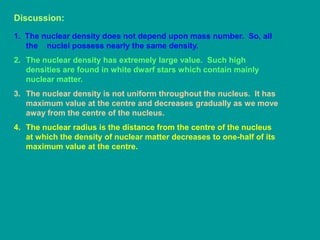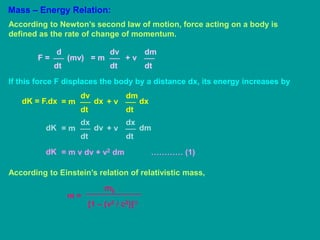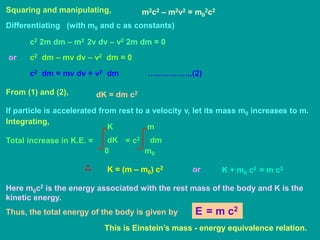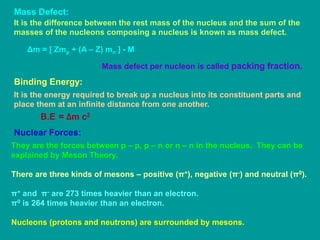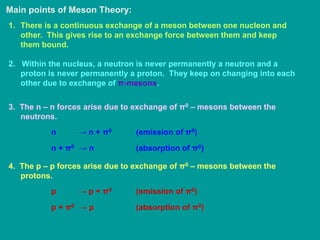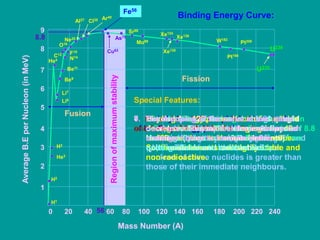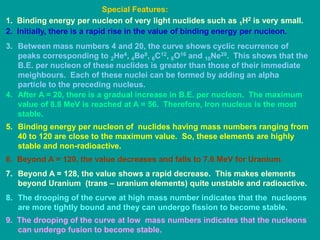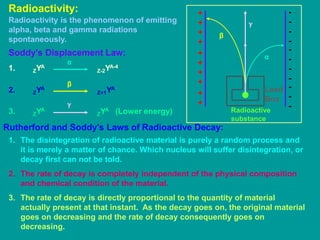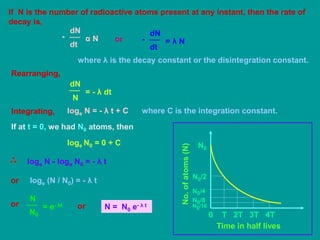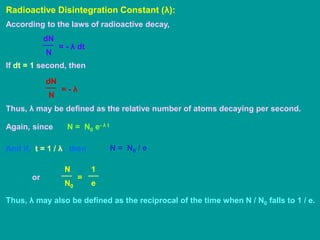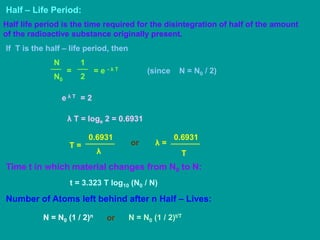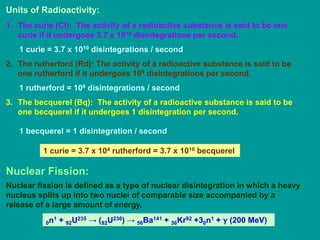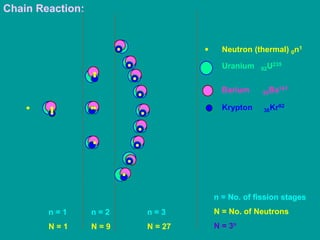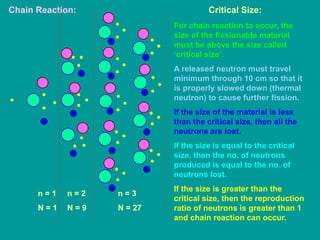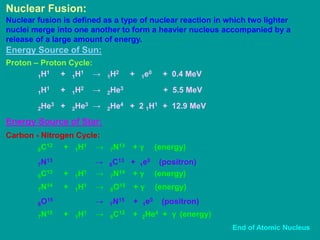1 von 24

### atomic_nucleus.ppt

• 1. ATOMIC NUCLEUS 1. Rutherford’s Alpha Scattering Experiment 2. Distance of Closest Approach (Nuclear Size) 3. Impact Parameter 4. Composition of Nucleus 5. Atomic Number, Mass Number and Atomic Mass Unit 6. Radius of the Nucleus and Nuclear Density 7. Mass Energy Relation and Mass Defect 8. Binding Energy and Binding Energy per Nucleon 9. Binding Energy Curve and Inferences 10.Nuclear Forces and Meson Theory 11.Radioactivity and Soddy’s Displacement Law 12.Rutherford and Soddy’s Laws of Radioactive Decay 13.Radioactive Disintegration Constant and Half-Life Period 14.Units of Radioactivity 15.Nuclear Fission and Fusion Created by C. Mani, Principal, K V No.1, Jalahalli (W), Bangalore
• 2. Rutherford’s Alpha Scattering Experiment + Lead Box Bi-214 or Radon α - Beam Thin Gold Foil ZnS Screen Gold Atom α - Beam Scattering angle (θ) No. of α-particles scattered (N) α α
• 3. Alpha – particle is a nucleus of helium atom carrying a charge of ‘+2e’ and mass equal to 4 times that of hydrogen atom. It travels with a speed nearly 104 m/s and is highly penetrating. Rutherford Experiment Geiger & Marsden Experiment Source of α-particle Radon 86Rn222 Bismuth 83Bi214 Speed of α-particle 104 m/s 1.6 x 107 m/s Thickness of Gold foil 10-6 m 2.1 x 10-7 m
• 4. S. No. Observation Conclusion 1 Most of the α-particles passed straight through the gold foil. It indicates that most of the space in an atom is empty. 2 Some of the α-particles were scattered by only small angles, of the order of a few degrees. α-particles being +vely charged and heavy compared to electron could only be deflected by heavy and positive region in an atom. It indicates that the positive charges and the most of the mass of the atom are concentrated at the centre called ‘nucleus’. 3 A few α-particles (1 in 9000) were deflected through large angles (even greater than 90°). Some of them even retraced their path. i.e. angle of deflection was 180°. α-particles which travel towards the nucleus directly get retarded due to Coulomb’s force of repulsion and ultimately comes to rest and then fly off in the opposite direction. N(θ) α 1 sin4(θ/2)
• 5. Distance of Closest Approach (Nuclear size): + r0 When the distance between α-particle and the nucleus is equal to the distance of the closest approach (r0), the α-particle comes to rest. At this point or distance, the kinetic energy of α-particle is completely converted into electric potential energy of the system. ½ mu2 = 1 4πε0 2 Ze2 r0 r0 = 1 4πε0 2 Ze2 ½ mu2
• 6. Impact Parameter (b): + r0 The perpendicular distance of the velocity vector of the α-particle from the centre of the nucleus when it is far away from the nucleus is known as impact parameter. θ b u b = 4πε0 Ze2 (½ mu2) cot (θ/2) i) For large value of b, cot θ/2 is large and θ, the scattering angle is small. i.e. α-particles travelling far away from the nucleus suffer small deflections. ii) For small value of b, cot θ/2 is also small and θ, the scattering angle is large. i.e. α-particles travelling close to the nucleus suffer large deflections. iii) For b = 0 i.e. α-particles directed towards the centre of the nucleus, cot θ/2 = 0 or θ/2 = 90° or θ = 180° The α-particles retrace their path.
• 7. Composition of Nucleus: Every atomic nucleus except that of Hydrogen has two types of particles – protons and neutrons. (Nucleus of Hydrogen contains only one proton) Proton is a fundamental particle with positive charge 1.6 x 10-19 C and mass 1.67 x 10-27 kg (1836 times heavier than an electron). Neutron is also a fundamental particle with no charge and mass 1.675 x 10-27 kg (1840 times heavier than an electron). Atomic Number (Z): The number of protons in a nucleus of an atom is called atomic number. Atomic Mass Number (A): The sum of number of protons and number of neutrons in a nucleus of an atom is called atomic mass number. A = Z + N Atomic Mass Unit (amu): Atomic Mass Unit (amu) is (1 / 12)th of mass of 1 atom of carbon. 1 amu = 1 12 12 x 6.023 x 1023 g = 1.66 x 10-27 kg
• 8. Size of Nucleus: Nucleus does not have a sharp or well-defined boundary. However, the radius of nucleus can be given by R = R0 A⅓ where R0 = 1.2 x 10-5 m is a constant which is the same for all nuclei and A is the mass number of the nucleus. Radius of nucleus ranges from 1 fm to 10 fm. Nuclear Volume, V = (4/3) π R3 = (4/3) π R0 3 A V α A Nucleus Density: Mass of nucleus, M = A amu = A x 1.66 x 10-27 kg Nuclear Volume, V = (4/3) π R3 = (4/3) π R0 3 A 4 3 22 7 x = x (1.2 x 10-15)3 A m3 = 7.24 x 10-45 A m3 Nucleus Density, ρ = M / V = 2.29 x 1017 kg / m3
• 9. Discussion: 1. The nuclear density does not depend upon mass number. So, all the nuclei possess nearly the same density. 2. The nuclear density has extremely large value. Such high densities are found in white dwarf stars which contain mainly nuclear matter. 3. The nuclear density is not uniform throughout the nucleus. It has maximum value at the centre and decreases gradually as we move away from the centre of the nucleus. 4. The nuclear radius is the distance from the centre of the nucleus at which the density of nuclear matter decreases to one-half of its maximum value at the centre.
• 10. Mass – Energy Relation: According to Newton’s second law of motion, force acting on a body is defined as the rate of change of momentum. d dt F = (mv) dv dt = m dm dt + v If this force F displaces the body by a distance dx, its energy increases by dv dt = m dK = F.dx dx dm dt + v dx dx dt = m dK dv dx dt + v dm = m v dv + v2 dm ………… (1) dK According to Einstein’s relation of relativistic mass, m = m0 [1 – (v2 / c2)]½
• 11. Squaring and manipulating, m2c2 – m2v2 = m0 2c2 Differentiating (with m0 and c as constants) c2 2m dm – m2 2v dv – v2 2m dm = 0 c2 dm – mv dv – v2 dm = 0 c2 dm = mv dv + v2 dm ……………..(2) From (1) and (2), dK = dm c2 If particle is accelerated from rest to a velocity v, let its mass m0 increases to m. Integrating, Total increase in K.E. = 0 K dK = c2 dm m0 m K = (m – m0) c2 or K + m0 c2 = m c2 Here m0c2 is the energy associated with the rest mass of the body and K is the kinetic energy. Thus, the total energy of the body is given by or E = m c2 This is Einstein’s mass - energy equivalence relation.
• 12. Mass Defect: It is the difference between the rest mass of the nucleus and the sum of the masses of the nucleons composing a nucleus is known as mass defect. Δm = [ Zmp + (A – Z) mn ] - M Mass defect per nucleon is called packing fraction. Binding Energy: It is the energy required to break up a nucleus into its constituent parts and place them at an infinite distance from one another. B.E = Δm c2 Nuclear Forces: They are the forces between p – p, p – n or n – n in the nucleus. They can be explained by Meson Theory. There are three kinds of mesons – positive (π+), negative (π-) and neutral (π0). π+ and π- are 273 times heavier than an electron. π0 is 264 times heavier than an electron. Nucleons (protons and neutrons) are surrounded by mesons.
• 13. Main points of Meson Theory: 1. There is a continuous exchange of a meson between one nucleon and other. This gives rise to an exchange force between them and keep them bound. 2. Within the nucleus, a neutron is never permanently a neutron and a proton is never permanently a proton. They keep on changing into each other due to exchange of π-mesons. 3. The n – n forces arise due to exchange of π0 – mesons between the neutrons. n → n + π0 (emission of π0) n + π0 → n (absorption of π0) 4. The p – p forces arise due to exchange of π0 – mesons between the protons. p → p + π0 (emission of π0) p + π0 → p (absorption of π0)
• 14. 5. The n – p forces arise due to exchange of π+ and π- mesons between the nucleons. n → p + π- (emission of π-) n + π+ → p (absorption of π+) p → n + π+ (emission of π+) p + π- → n (absorption of π-) 6. The time involved in such an exchange is so small that the free meson particles cannot be detected as such. Binding Energy per Nucleon: It is the binding energy divided by total number of nucleons. It is denoted by B B = B.E / Nucleon = Δm c2 / A
• 15. 0 20 40 60 80 100 120 140 160 180 200 220 240 Mass Number (A) Average B.E per Nucleon (in MeV) 6 7 5 1 4 8 3 9 2 8.8 Region of maximum stability Fission Fusion Binding Energy Curve: Special Features: 1. Binding energy per nucleon of very light nuclides such as 1H2 is very small. 2. Initially, there is a rapid rise in the value of binding energy per nucleon. 3. Between mass numbers 4 and 20, the curve shows cyclic recurrence of peaks corresponding to 2He4, 4Be8, 6C12, 8O16 and 10Ne20. This shows that the B.E. per nucleon of these nuclides is greater than those of their immediate neighbours. 4. After A = 20, there is a gradual increase in B.E. per nucleon. The maximum value of 8.8 MeV is reached at A = 56. Therefore, Iron nucleus is the most stable. 5. Binding energy per nucleon of nuclides having mass numbers ranging from 40 to 120 are close to the maximum value. So, these elements are highly stable and non-radioactive. 6. Beyond A = 120, the value decreases and falls to 7.6 MeV for Uranium. 7. Beyond A = 128, the value shows a rapid decrease. This makes elements beyond Uranium (trans – uranium elements) quite unstable and radioactive. 8. The drooping of the curve at high mass number indicates that the nucleons are more tightly bound and they can undergo fission to become stable. 9. The drooping of the curve at low mass numbers indicates that the nucleons can undergo fusion to become stable. 56 Li7 Li6 He4 Be11 C12 N14 F19 Be9 O16 Ne20 Al27 Cl35 Ar40 Fe56 Mo98 Xe124 Xe136 Xe130 As75 Sr86 Cu63 W182 Pt208 U235 U238 Pt194 H1 H2 H3 He3
• 16. 1. Binding energy per nucleon of very light nuclides such as 1H2 is very small. 2. Initially, there is a rapid rise in the value of binding energy per nucleon. 3. Between mass numbers 4 and 20, the curve shows cyclic recurrence of peaks corresponding to 2He4, 4Be8, 6C12, 8O16 and 10Ne20. This shows that the B.E. per nucleon of these nuclides is greater than those of their immediate meighbours. Each of these nuclei can be formed by adding an alpha particle to the preceding nucleus. 4. After A = 20, there is a gradual increase in B.E. per nucleon. The maximum value of 8.8 MeV is reached at A = 56. Therefore, Iron nucleus is the most stable. 5. Binding energy per nucleon of nuclides having mass numbers ranging from 40 to 120 are close to the maximum value. So, these elements are highly stable and non-radioactive. 6. Beyond A = 120, the value decreases and falls to 7.6 MeV for Uranium. 7. Beyond A = 128, the value shows a rapid decrease. This makes elements beyond Uranium (trans – uranium elements) quite unstable and radioactive. 8. The drooping of the curve at high mass number indicates that the nucleons are more tightly bound and they can undergo fission to become stable. 9. The drooping of the curve at low mass numbers indicates that the nucleons can undergo fusion to become stable. Special Features:
• 17. Radioactivity: Lead Box Radioactive substance α β γ - - - - - - - - - - - + + + + + + + + + + Radioactivity is the phenomenon of emitting alpha, beta and gamma radiations spontaneously. Soddy’s Displacement Law: 1. ZYA Z-2YA-4 α 2. ZYA Z+1YA β 3. ZYA ZYA (Lower energy) γ Rutherford and Soddy’s Laws of Radioactive Decay: 1. The disintegration of radioactive material is purely a random process and it is merely a matter of chance. Which nucleus will suffer disintegration, or decay first can not be told. 2. The rate of decay is completely independent of the physical composition and chemical condition of the material. 3. The rate of decay is directly proportional to the quantity of material actually present at that instant. As the decay goes on, the original material goes on decreasing and the rate of decay consequently goes on decreasing.
• 18. If N is the number of radioactive atoms present at any instant, then the rate of decay is, dt dN - α N or dN dt - = λ N where λ is the decay constant or the disintegration constant. Rearranging, N dN = - λ dt Integrating, loge N = - λ t + C where C is the integration constant. If at t = 0, we had N0 atoms, then loge N0 = 0 + C loge N - loge N0 = - λ t or loge (N / N0) = - λ t or N = e- λt N0 or N = N0 e- λ t No. of atoms (N) N0 N0/2 N0/4 N0/8 N0/16 Time in half lives 0 T 2T 3T 4T
• 19. Radioactive Disintegration Constant (λ): According to the laws of radioactive decay, N dN = - λ dt If dt = 1 second, then N dN = - λ Thus, λ may be defined as the relative number of atoms decaying per second. Again, since N = N0 e- λ t And if, t = 1 / λ, then N = N0 / e or N0 N = e 1 Thus, λ may also be defined as the reciprocal of the time when N / N0 falls to 1 / e.
• 20. Half – Life Period: Half life period is the time required for the disintegration of half of the amount of the radioactive substance originally present. If T is the half – life period, then N0 N = 2 1 = e - λ T e λ T = 2 (since N = N0 / 2) λ T = loge 2 = 0.6931 T = λ 0.6931 T λ = 0.6931 or Time t in which material changes from N0 to N: t = 3.323 T log10 (N0 / N) Number of Atoms left behind after n Half – Lives: N = N0 (1 / 2)t/T N = N0 (1 / 2)n or
• 21. Units of Radioactivity: 1. The curie (Ci): The activity of a radioactive substance is said to be one curie if it undergoes 3.7 x 1010 disintegrations per second. 1 curie = 3.7 x 1010 disintegrations / second 2. The rutherford (Rd): The activity of a radioactive substance is said to be one rutherford if it undergoes 106 disintegrations per second. 1 rutherford = 106 disintegrations / second 3. The becquerel (Bq): The activity of a radioactive substance is said to be one becquerel if it undergoes 1 disintegration per second. 1 becquerel = 1 disintegration / second 1 curie = 3.7 x 104 rutherford = 3.7 x 1010 becquerel Nuclear Fission: Nuclear fission is defined as a type of nuclear disintegration in which a heavy nucleus splits up into two nuclei of comparable size accompanied by a release of a large amount of energy. 0n1 + 92U235 → (92U236) → 56Ba141 + 36Kr92 +30n1 + γ (200 MeV)
• 22. Chain Reaction: n = 1 N = 1 n = 2 N = 9 n = 3 N = 27 Neutron (thermal) 0n1 Uranium 92U235 Barium 56Ba141 Krypton 36Kr92 n = No. of fission stages N = No. of Neutrons N = 3n
• 23. Chain Reaction: n = 1 N = 1 n = 2 N = 9 n = 3 N = 27 Critical Size: For chain reaction to occur, the size of the fissionable material must be above the size called ‘critical size’. A released neutron must travel minimum through 10 cm so that it is properly slowed down (thermal neutron) to cause further fission. If the size of the material is less than the critical size, then all the neutrons are lost. If the size is equal to the critical size, then the no. of neutrons produced is equal to the no. of neutrons lost. If the size is greater than the critical size, then the reproduction ratio of neutrons is greater than 1 and chain reaction can occur.
• 24. Nuclear Fusion: Nuclear fusion is defined as a type of nuclear reaction in which two lighter nuclei merge into one another to form a heavier nucleus accompanied by a release of a large amount of energy. Energy Source of Sun: Proton – Proton Cycle: 1H1 + 1H1 → 1H2 + 1e0 + 0.4 MeV 1H1 + 1H2 → 2He3 + 5.5 MeV 2He3 + 2He3 → 2He4 + 2 1H1 + 12.9 MeV Carbon - Nitrogen Cycle: 6C12 + 1H1 → 7N13 + γ (energy) 7N13 → 6C13 + 1e0 (positron) Energy Source of Star: 6C13 + 1H1 → 7N14 + γ (energy) 7N14 + 1H1 → 8O15 + γ (energy) 8O15 → 7N15 + 1e0 (positron) 7N15 + 1H1 → 6C12 + 2He4 + γ (energy) End of Atomic Nucleus